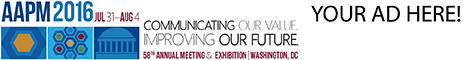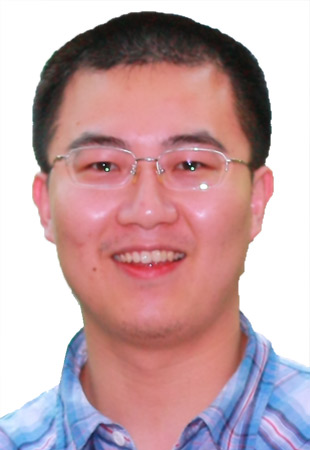# Program Information

## Explore the Sensitive of the Planar Quality Assurance to the MLC Error with Different Beam Complexity in Intensity-Modulate Radiation TherapyJ Wang

## Presentations

#### SU-E-T-418 (Sunday, July 12, 2015) 3:00 PM - 6:00 PM Room: Exhibit Hall

Purpose: The purpose of this study is to investigate the sensitivity of the planar quality assurance to MLC errors with different beam complexities in intensity-modulate radiation therapy.
Methods: sixteen patients’ planar quality assurance (QA) plans in our institution were enrolled in this study, including 10 dynamic MLC (DMLC) IMRT plans measured by Portal Dosimetry and 6 static MLC (SMLC) IMRT plans measured by Mapcheck. The gamma pass rate was calculated using vender’s software. The field numbers were 74 and 40 for DMLC and SMLC, respectively. A random error was generated and introduced to these fields. The modified gamma pass rate was calculated by comparing the original measured fluence and modified fields’ fluence. A decreasing gamma pass rate was acquired using the original gamma pass rate minus the modified gamma pass rate. Eight complexity scores were calculated in MATLAB based on the fluence and MLC sequence of these fields. The complexity scores include fractal dimension, monitor unit of field, modulation index, fluence map complexity, weighted average of field area, weighted average of field perimeter, and small aperture ratio ( <5cm² and <50cm²). The Spearman's rank correlation coefficient was implemented to analyze the correlation between these scores and decreasing gamma rate .
Results: The relation between the decreasing gamma pass rate and field complexity was insignificant for most complexity scores. The most significant complexity score was fluence map complexity for SMLC, which have ρ=0.4274 (p-value=0.0063). For DMLC, the most significant complex score was fractal dimension, which have ρ=-0.3068 (p-value=0.0081).
Conclusions: According to the primarily result of this study, the sensitivity gamma pass rate was not strongly relate to the field complexity.

Contact Email: